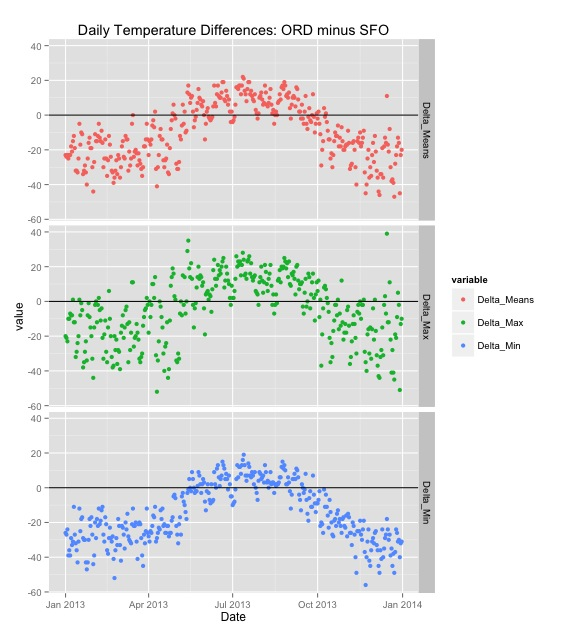View on GitHub

# weatherData

## An R package that fetches Weather data from websites

Download this project as a .zip file Download this project as a tar.gz file

`weatherData` is a collection of functions that will fetch weather (Temperature, Pressure, Humidity etc.) data from the Web for you as clean data frame.

### Example showing the use of getWeatherForYear()

``````library(weatherData)
``````

#### Usage: getWeatherForYear("station_id", "YYYY")

First, we get two data frames, with one year's worth of temperature data in each. (365 rows each.) Note that `getWeatherForYear` is a function in the `weatherData` package.

``````city1 <- "ORD"
city2 <- "SFO"
df1 <- getWeatherForYear(city1, 2013)
df2 <- getWeatherForYear(city2, 2013)
``````

Given two dataframes that contain daily temperatures (mean, min and max), we are simply taking the vectorized differences and creating another data frame.

``````getDailyDifferences <- function(df1, df2){
Delta_Means <- df1\$Mean_TemperatureF - df2\$Mean_TemperatureF
Delta_Max <- df1\$Max_TemperatureF - df2\$Max_TemperatureF
Delta_Min <- df1\$Min_TemperatureF - df2\$Min_TemperatureF

diff_df <- data.frame(Date=df1\$Date, Delta_Means, Delta_Max, Delta_Min)
return(diff_df)
}
``````

Next, let's write a small function using `ggplot` to plot the daily differences. We will use 3 facets, one each for Mean, Max and Min difference.

``````plotDifferences <- function (differences, city1, city2) {
library(reshape2)
m.diff <- melt(differences, id.vars=c("Date"))
p <- ggplot(m.diff, aes(x=Date, y=value)) + geom_point(aes(color=variable)) +
facet_grid(variable ~ .) +geom_hline(yintercept=0)
p <- p + labs(title=paste0("Daily Temperature Differences: ", city1, " minus ",city2))
print(p)
}
``````

With these two functions in place, given any two cities, we can create the difference data frame and plot the differences. Let's try it for Chicago and San Francisco.

``````differences<- getDailyDifferences(df1, df2)
plotDifferences(differences, city1, city2)
``````

And here's the resulting plot:<< Back to Examples